## MODERN PRACTICE OF THE ELECTRIC TELEGRAPH

### TESTING TELEGRAPH LINES

114. Interruption and interferences from various causes are constantly occurring upon telegraph lines, and one of the most important of an operator's duties is to be able to discover promptly the nature and location of a fault, that measures may immediately be taken for its removal. This is done by an investigation called testing. The apparatus and methods now in general use in this country are of a somewhat primitive nature, but the improved modes of testing which have long been employed in Europe are gradually becoming appreciated here, and as these are based on sound scientific principles, it is to be hoped that they will soon supersede the imperfect ones heretofore employed.

115. The principal interruptions to which a telegraphic circuit are liable may be summed up as follows :

Disconnection.--- The continuity of the circuit is broken, so that no current passes over the line.

Partial Disconnection.--- This is usually caused by rusty and unsoldered joints in the line, or by loose screw connections in offices or about switches, which offer great resistance to the passage of the current.

Escape or leakage of current from the line to the ground, caused by defective insulation, or contact with trees, &c. When an escape is sufficient to entirely prevent the working of the line it is called a ``ground.''

Cross.--- When two wires are in contact, so that one cannot be worked without interfering with the other.

Weather Cross.--- When a portion of the current from one wire leaks into others upon the same poles, through defective insulation. The effect is similar to that of a cross, but much less strongly marked. This is often improperly called sympathy, or induction.

Defective Ground Connection.--- It sometimes happens that the ground wire, or ground plate, at a terminal station, is defective. The effect of this is to make the wires connected with it appear as if in contact, or crossed. This difficulty is often caused by the removal of a meter in offices where a gas pipe is used as a ground connection for several lines.

116. Testing for Disconnection.--- If the circuit is broken at any point the relays will all remain open. The operator at each way station should immediately proceed to test the wire by connecting his ground wire, first on one side of the instruments and then on the other. If either connection closes the line circuit, the interruption is on that side, as the circuit of the opposite main battery is completed through the ground, in place of the broken wire. If the ground wire gives no circuit either way, it is probable that the interruption is in the office, or that the ground connection is defective. Each operator should always first make sure that the fault is not in or about his own office. Having ascertained the direction in which the difficulty lies, he should at once report the state of the case to the terminal station at the opposite end.

Fig. 45 represents a line with four stations, A, B, C and D. Suppose the wire broken at F. By connecting the ground wires at B and C, as shown, two distinct circuits are formed. A can work with B, and C with D, showing that the fault is between B and C.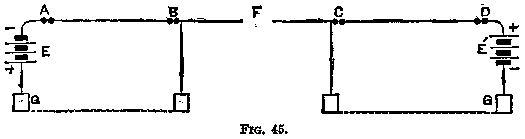Disconnection is usually caused by the breaking of the line wire, or by a key carelessly left open. Some other causes are wires loose in their binding screws, or defective switches, or the fine wire in or about the relay may be broken. The latter is sometimes burned in two by atmospheric electricity.

117. Partial Disconnection.--- It is rather difficult to discover this fault by the ordinary relay tests. It is frequently of an intermittent character, and requires to be very carefully tested for. In the latter case, the best plan is to cross connect, or interchange the defective wire with a good one at the terminal, and also one other station, as in Fig. 46. Suppose the fault is at F, on No. 2 wire ; by cross connection at A and B, as shown, the fault will shift to No. 1 circuit, showing that it is between those points. If it were beyond B, it would remain on No. 2 circuit. In this case, let the wires be put straight at B, and cross connected at C, and so on, station by station. When the fault is passed it shifts to the other circuit, and will therefore be found between the two last stations.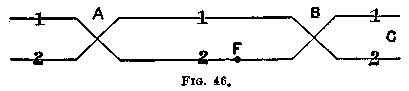118. To Test for an Escape.--- Call the stations up in rotation, beginning with the one farthest off, and have them open key for a minute or two. When a station beyond the escape is open, more or less current will still pass out to the line through the relay, returning through the ground from the fault.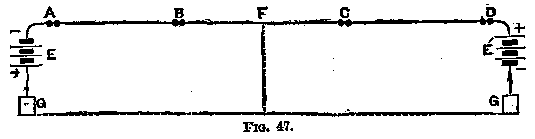Suppose A (Fig. 47) is testing. When the circuit is open at C or D a current will pass from E through the fault, F, which will be interrupted when B opens, showing the fault is between B and C.

119. Testing for Grounds.--- A ground is tested for in a similar manner. The operator at a way station can ascertain which side of him the ground is situated, from the fact that it cuts off or greatly weakens the main battery current from that direction, when tested with a ground wire by means of the finger or tongue.

Telegraph lines are very liable to be grounded by the action of atmospheric electricity upon the lightning arresters. These should be carefully looked after at frequent intervals, especially where exposed to dampness, as in cable boxes.

120. Testing for Crosses.--- In case a cross is suspected between two wires, say Nos. 1 and 2, instruct the most distant station to open one wire, preferably the through wire, or No. 1, and ``send dots'' upon the other. Open No. 2 at your own station, and if the dots sent on No. 2 at the distant station are received on No. 1, the wires are crossed. Care must, of course, be taken not to be deceived by the leakage from one wire to another, caused by defective insulation. If the wires are in actual contact, the signals received upon No. 2 wire will be nearly or quite as strong as if received upon No. 1.

Next, instruct the distant station to leave No. 1 open, and open it also at your own station. No. 2 will now be free from interference, and the stations upon it may be signalled without difficulty. Call them in regular succession, commencing at the farthest end of the line, and instruct each one in turn to send dots on No. 2. If the dots are received on both wires the cross is between you and the station sending ; but if upon No. 2 only, it is beyond that station. It is better that each operator, while sending dots, should open the other wire, if practicable.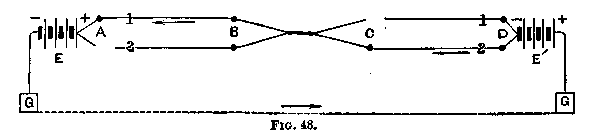The principle of this test will be understood by reference to Figs. 48 and 49, which represent a two wire line, with four stations, A, B, C and D, the wires being ``crossed'' between B and C. The operator testing for the cross is supposed to be at A. In Fig. 48 station C has No. 1 open, and station A has No. 2 open. If C sends dots on No. 2 the circuit will shift to No. 1, at the cross, as shown by the arrows, and the dots will come on No. 1 instrument at A, showing that the cross is between A and C. In case C were unable to open No. 1 the effect would evidently be the same, provided it remains open at D.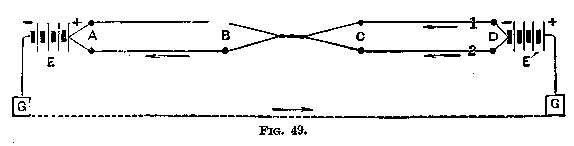Now let C close both wires, and B open No. 1, and write dots on 2 (Fig. 49). If No. 2 be open at A, B will be unable to work in this case, as both wires are open, one at A and the other at B. With both wires closed at A, B's dots will come on No. 2, the current from F passing over both wires to the cross, and from thence on No. 2, No. 1 being open, as shown in the figure. Thus the fault is located between B and C.

In large offices, where there are a considerable number of wires, it will often be found a much more convenient and expeditious method of testing for crosses for the operator to station himself at the switch with a single instrument, which can be placed at pleasure on any wire, for the purpose of communicating with different stations. When any station is ``sending dots'' the testing operator can feel them by placing a finger upon the ground wire, and another upon the proper line wire at the switch. The principle involved is of course the same as in the method first described. In wet weather, however, testing by the sense of feeling is attended with much uncertainty, as it is impossible to distinguish between the effect of a metallic cross, or actual contact, and the leakage arising from bad insulation.

121. It would be difficult to specify all the minor interruptions that are liable to occur in and about telegraph offices, or on the lines ; the operator will therefore, in many cases, be obliged to depend upon his own ingenuity for the best method of testing applicable to each particular case. By carefully studying, however, the principles heretofore explained, the intelligent telegrapher will usually be able to cope with any difficulty that may chance to arise in the ordinary service of the lines.

122. Testing with the Galvanometer and Resistance Coils.--- In the more accurate and scientific modes of testing, which have been for some years employed upon the European lines, the instruments used are the differential galvanometer and a set of standard resistance coils (44).

The arrangement of the connections will be understood by reference to Fig. 50.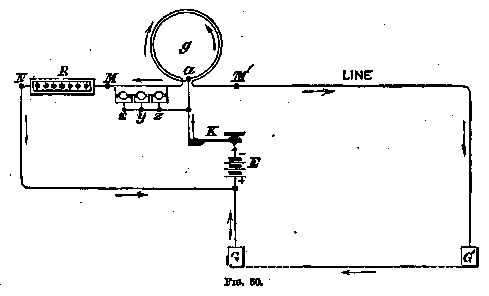The galvanometer coils are wound with two wires of the same length and resistance, insulated from each other with the utmost care. The needle is, therefore, surrounded by an equal number of convolutions of each wire, which are also equi-distant from it.

The inner end of one coil surrounding the galvanometer, g, is joined to the outer end of the other at a, and a key, K, attached to this junction, when depressed, forms the connection with the testing battery, E. The other ends of the coils run to binding screws, M M', for the convenient attachment of lines to be tested. The principle upon which the action of the instrument depends is the following:

When the battery is connected by depressing the key the current divides into two equal portions at a, one flowing from a to M, tending to deflect the needle to the left, and the other from a to M', tending to deflect it to the right ; but as long as the two currents are of the same strength they balance each other, and the needle remains at rest. Suppose that the terminal M' is connected to a telegraph line whose remote end is to ground, and the terminal M is connected through the resistance coils, R, to the ground likewise, as shown in Fig. 50. We have already seen (102) that the strength of an electric current is in all cases equal to the electro-motive force divided by the resistance. Therefore, if in this case we let

```E = electro-motive force of battery,
l = resistance of line wire,
g = resistance of galvanometer coil,
r = resistance coils in circuit,
```

the current in the two circuits surrounding the needle will be

```E             E
-------  and  -------
g + l'        g + r
```

If therefore, the resistance coils in circuit be varied until r = l, the needle will remain unaffected. As the earth offers no appreciable resistance to the passage of the current, the resistance of the line l will be accurately represented by the amount of resistance interposed at r, in order to bring the needle to zero. The value of the above equation will obviously not be affected by any change in the value of E.

123. The resistance coils, R, accompanying the galvanometer, are so arranged as to be adjustable to any required resistance, from 1 ohm up to 10,000. For the measurement of still higher resistances one coil of the galvanometer is provided with three ``shunts,'' or branch circuits, x, y, z, having resistances respectively equal to g/9, g/99, and g/999; therefore, if x be connected, 1/10 of the current will pass through g, and 9/10 through one wire of the galvanometer, when connected, and by this means the instrument may be made to measure any resistance, from 0.001 up to 10,000,000 ohms.

124. Testing for the Distance of Faults.--- The principle upon which the methods of distance testing are founded is that of finding the resistance of the line wire between the testing station and the fault by means of the apparatus described. When the line is broken at any point one of the following four cases generally occurs :

1. Line wire broken, giving full, or nearly full, ground connection.

2. Line wire unbroken, but gives nearly enough escape to ground to make signals imperceptible.

3. Line wire broken, without making contact with earth.

4. A cross between two wires, so that signals sent on one are communicated to both.

125. It is very essential that the resistance of each circuit should be frequently measured and recorded, so that when a fault occurs the actual resistance per mile of the line may be known. If the broken line gives a full ground, its resistance divided by the resistance per mile at once gives the distance of the break from the testing station ; and if the distant station obtains a corresponding result, the confirmation is complete. Thus, in a line of 100 miles in length, if the tests from the two extremities indicate distances of 45 and 55 miles, respectively, the locality of the interruption is clearly indicated. As the fault, however, usually gives a very considerable resistance at the point where the line is in contact with the earth, and the sum of the two resistances, measured from stations at the opposite ends of the line, greatly exceeds the resistance of the line itself when perfect, it is usual in such cases to estimate the fault midway between the two points indicated. Thus, when the respective resistances indicate 86 and 26 miles, the sum of these exceeds 100 miles by 12, and therefore half this excess, or 6, is deducted from each of the measures.

126. When the line is unbroken, but shows a heavy escape or partial ground, sufficient to weaken signals, two or three different methods are available for determining its locality. The first plan is that of direct measurement, alternately from each end, the distant end at the same time being insulated, or, in other words, left open, in the manner explained in the last paragraph. In this case the resistance of the fault is measured twice over, and is roughly allowed for by the method of calculation above given.

127. The Loop Test.--- A second and more accurate method, which gives a measure entirely independent of the resistance of the fault itself, is known as the loop test. It is only available, however, in cases where there are two or more parallel wires on the same route. In order to make this test, let the operator proceed as follows :

Make the length to be tested as short as possible, and have all the instruments in circuit taken out. Select a good wire, similar, if possible, to the one it is required to test. Both these wires must then be connected together in a loop, at the nearest available station beyond the fault, without ground connection. The resistance of the faulty wire, when perfect, must be ascertained. This may be taken from previous records, or it may be found thus :

Connect one end of the loop to the + pole of the battery E, and the other to one of the galvanometer wires at M'. Connect also the + pole of the battery with the resistance coils, R, at N, and the -pole of the battery to the key, K, and common terminal, a, of the galvanometer. Connect the remaining galvanometer wire with the resistance coils at M (Fig. 51).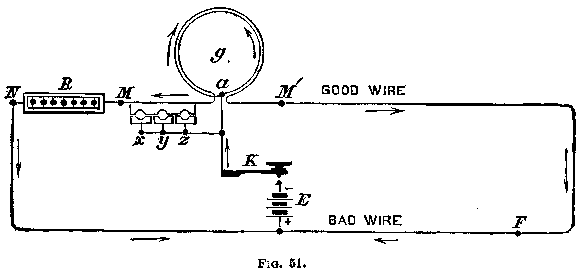Having ascertained the resistance of the loop, arrange the connections as shown in Fig. 52.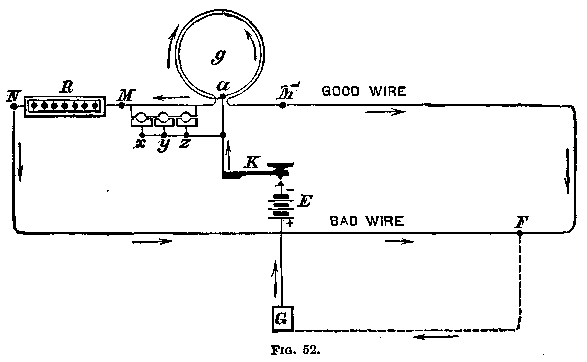Upon depressing the key, K, the battery current will flow through M and R, and also through the loop. The resistance required at R to balance the needle will be equal to the sum of the resistance of the two lines. Although there is a partial ground at F it will not affect the measurement, as there is no other ground in circuit.

Connect the + pole of the battery E to ground, and connect the -pole to the key K. Connect the perfect wire of the loop to one of the galvanometer wires at M', and the faulty wire to the other galvanometer wire at M, interposing the resistance coils R. Then the key K is depressed the current from the battery E flows into both lines simultaneously, passing to the ground through the fault at F. By adding resistance at R, so as to bring the needle to zero, the resistance a N F will be made equal to a M' F.

The resistance thus added, deducted from the total resistance of the loop, previously ascertained, and divided by two, is the resistance of the line between N and M.

Thus, if the resistance of the loop be 1,000 ohms, and 100 ohms have been added to the defective wire to balance the needle---

```1000 - 100
Then ------------ = 450 ohms, the resistance of the wire
2
between the resistance coils R and the fault.
Let M' F = x, N F = y.
Then x + y = L, the resistance of the loop.
As R + y = x, or R + N F = M' F.
L - R
Therefore, y = -------
2
```

Suppose that the loop of 1,000 ohms measures 120 miles, then by proportion,

```If 1,000 ohms = 120 miles, 450 ohms = 54 miles.
```

When an instrument or section of small wire is included in the circuit, allowance must be made for their resistance. It is a great assistance in these tests to know from previous records the exact resistance of every section of the line.

128. Blavier's Formula for Locating an Escape.--- Where there is but one wire the following method may be employed. Three tests have to be taken for the operation, viz :

```Let R = resistance of the line before it was defective.  This must
be obtained from previous records.
``  S = resistance of the line when grounded at the distant end.
``  T = resistance of the line when disconnected at the distant end.
```

Multiply S by S and T by R, and add the products together ; subtract from this amount T times S, and also R times S. Subtract the square root of the remainder from S ; the remainder will give the resistance, x or the distance of the fault from the testing station.

This process appears complicated but is in reality very simple. For example, suppose the line 100 units long, and the fault 68 units distant, and the resistance of the fault 96 units, as shown in Fig. 53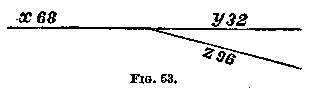```Then R = x + y        = 68 + 32         = 100
y x z            32 x 96
*S = x + -------  = 68 + ---------  =  92
y + z            32 + 96
T = x + z        = 68 + 96         = 164
```

// footnote

* See note, (sec.) 104.

// end footnote

We shall, however, have obtained these resistances by measurement and not by calculation. We therefore have :

```S x S =  92 x  92 =  8464  \
T x R = 164 x 100 = 16400   > 24864
T x S = 164 x  92 = 15088  \
R x S = 100 x  92 =  9200   > 24288
-------
576
```

And the square root of 576 is 24; which deducted, S = 92, gives 68 as the resistance of x, or the distance of the fault from the testing station.

The distance, x being known, the others are obtained with ease ; for R -68 gives y, the distance from the opposite end; and T -68 gives z, or the resistance of the fault itself. This test should be taken from both ends of the line, if possible. In the above calculation the resistance of the fault is supposed to remain constant during the measurements ; but as this is not often the case in practice, the average of several measurements should be taken.

129. To Find the Distance of a Cross.--- The two wires in contact form a loop, provided they are clean, and are twisted together, so that the contact offers no appreciable resistance. In such a case open both wires at the nearest station beyond, and test the resistance of the loop. Half this resistance will be the resistance of the wire between the galvanometer and the fault, and from this the distance can be calculated, as before explained (127). All relays in circuit must be taken out, or the proper allowance made for their resistance.

As it is difficult to tell with certainty whether the cross offers resistance of not, it is a better plan to test it as a ground. Test each wire in turn by the loop method (127), grounding the wire at both ends. The wire tested will then make a ground through the other wire at the point of contact, and the location of the latter may be readily ascertained.

Second Method.*--- Suppose two wires, A and B, touch one another at the point F. Connect A to the zinc of the testing battery, leaving it open at the remote end ; it will then serve as a battery wire between the battery and the fault (F). Ground B at the distant end, and connect it to one coil of the differential galvanometer at the testing station. Put the other wire of the galvanometer to ground. The current of the battery will pass along the wire A and divide at F, one portion going to ground at the distant end of B, and reaching the galvanometer through the wire connected with the ground, the other portion returning to the galvanometer through the nearer portion of B. If the cross is exactly in the centre of B the needle will not move, as the two currents will balance each other. If one section of B is longer than the other, the resistance added to the shorter section to balance the needle will show the difference in the resistance of the two sections.

// footnote

* Culley's Handbook, 3d edition, page 279

// end footnote

```Let L = the total resistance of B.
`` x = resistance of the shorter portion.
`` L - x = ``       ``   longer    ``
`` R = resistance added to shorter portion.
L - R
Then x = -------
2
```

130. Advantages of Testing by Measurement.--- The testing of lines by actual measurement lies at the very foundation of all efforts to improve the working of our telegraphic system. The insulation resistance of each of the principal circuits should be measured every morning, and a record of the results kept for reference. In England the standard of insulation is 1,000,000 ohms per mile in the worst of weather. Therefore, a line of 200 miles should not give less than

```1,000,000
----------- = 5,000
200
```

ohms. If it gives less than this the low resistance is due to defective insulation. The line should, in that case, be tested in many separate sections, either from the terminal office or by a visit to each section. If the resistance per mile is the same for each section, the fault is probably owing to the nature of the insulation ; but if, as is usually the case, some sections are very much worse than others, the trouble will be found in contact with trees, broken insulators, and the like. A visit to the faulty locality will disclose the cause of the evil.

131. In comparing the insulation of line wires of different lengths, the insulation per mile must be ascertained, otherwise the longest wire will appear the worst ; therefore, multiply the insulation test in ohms by the length of the wire in miles. If the insulation is uniformly good throughout the circuit tested, the leakage will increase in direct proportion to the length of the wire, irrespective of its thickness or conducting power, for the resistance of the wire is very small in comparison with the insulators, and need not be taken into account.

The following example from Culley's work will illustrate this. The figures given are the results of an actual test :

```The wire A had a leakage equal to.......  29
``    B   ``     ``      ``   .......  30
``    C   ``     ``      ``   .......  50
-----
Total leakage........................ 109```

The three wires, when connected together at the testing end and left open at the distant end, gave a combined leakage of 110.

When connected so as to form a continuous wire, open at the distant end, the leakage was still 110. The experiment was repeated, and extended to other wires, with the same result. In this case the resistance of the insulators was very great compared with that of the wire---as much as two million ohms per mile. But on a wet day three similar wires, whose respective leakages were 196, 185 and 141, making a total of 552, when looped in a continuous line, as in the second case above, gave a test of only 476, the distant portion of the wire being in reality tested by a current weakened by the leakage in the nearer portions.

132. Testing for Conductivity Resistance.--- The metallic resistance of the line wires should be occasionally tested in sections, in the finest weather. The resistance should be uniformly in proportion to the length of the wire. If any section discloses an unusually high resistance per mile, it is very probable that there are rusty, unsoldered joints in the line, or that the ground connections are defective. It is difficult for those who have not tried it to believe the vast improvement that may be made in any line in a few days by actual measurement, and an inspection of the sections which give indications of being defective.

It is not an uncommon occurrence to find that a single unsoldered joint in galvanized iron wire, which appears perfectly firm and sound, will give a resistance, when tested by the galvanometer, equal to many miles of line. A line containing many bad joints will frequently work better in wet than in dry weather, as the moisture increases the conductivity of the oxide between the wires at the joints.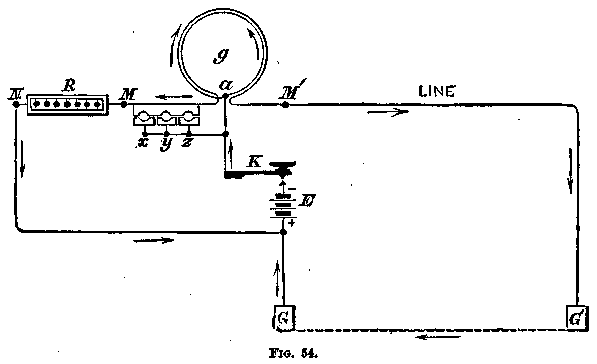In testing for conductivity, with the distant end of the wire to ground, as in Fig. 54, the result is sometimes interfered with by earth currents. It is therefore better, when practicable, to use the loop method, by connecting the wire to be measured in a loop with another wire of known resistance. Unless this test is made in fine weather, however, the leakage from one wire to the other will decrease the resistance of the loop. The battery must also be insulated from the earth, otherwise the leakage at each insulator will decrease the apparent resistance, especially if the insulation is defective. For instance, two wires on the same poles, disconnected and looped at the distant end, had a resistance of 6,475 ohms when the battery was entirely disconnected from the earth. Upon putting the zinc pole of the battery and the line attached to it to earth, the apparent resistance fell to 5,250 ohms. The insulation resistance, with one wire disconnected, was 9,250 ohms, the weather being damp.